Test: Laplace Transform- 3

# Test: Laplace Transform- 3

Test Description

## 10 Questions MCQ Test GATE ECE (Electronics) 2023 Mock Test Series | Test: Laplace Transform- 3

Test: Laplace Transform- 3 for Electronics and Communication Engineering (ECE) 2023 is part of GATE ECE (Electronics) 2023 Mock Test Series preparation. The Test: Laplace Transform- 3 questions and answers have been prepared according to the Electronics and Communication Engineering (ECE) exam syllabus.The Test: Laplace Transform- 3 MCQs are made for Electronics and Communication Engineering (ECE) 2023 Exam. Find important definitions, questions, notes, meanings, examples, exercises, MCQs and online tests for Test: Laplace Transform- 3 below.
Solutions of Test: Laplace Transform- 3 questions in English are available as part of our GATE ECE (Electronics) 2023 Mock Test Series for Electronics and Communication Engineering (ECE) & Test: Laplace Transform- 3 solutions in Hindi for GATE ECE (Electronics) 2023 Mock Test Series course. Download more important topics, notes, lectures and mock test series for Electronics and Communication Engineering (ECE) Exam by signing up for free. Attempt Test: Laplace Transform- 3 | 10 questions in 30 minutes | Mock test for Electronics and Communication Engineering (ECE) preparation | Free important questions MCQ to study GATE ECE (Electronics) 2023 Mock Test Series for Electronics and Communication Engineering (ECE) Exam | Download free PDF with solutions
 1 Crore+ students have signed up on EduRev. Have you?
Test: Laplace Transform- 3 - Question 1

### Determine the time signal x(t) corresponding to given X (s) and choose correct option. Q.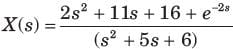Detailed Solution for Test: Laplace Transform- 3 - Question 1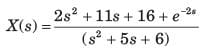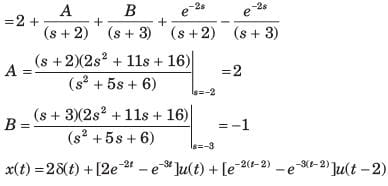Test: Laplace Transform- 3 - Question 2

### Determine the time signal x(t) corresponding to given X (s) and choose correct option. Q.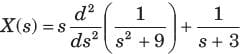Detailed Solution for Test: Laplace Transform- 3 - Question 2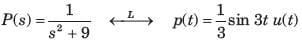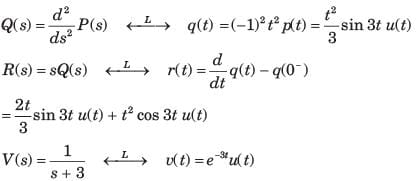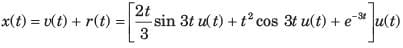Test: Laplace Transform- 3 - Question 3

### Determine the time signal x(t) corresponding to given X (s) and choose correct option. Q.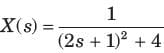Detailed Solution for Test: Laplace Transform- 3 - Question 3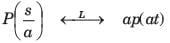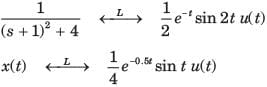Test: Laplace Transform- 3 - Question 4

Determine the time signal x(t) corresponding to given X (s) and choose correct option.
Q.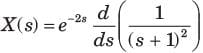Detailed Solution for Test: Laplace Transform- 3 - Question 4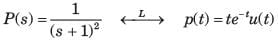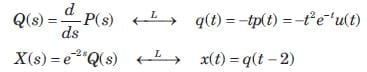x(t) =  -( t - 2) 2e-(t-2) u( t - 2)

Test: Laplace Transform- 3 - Question 5

Given the transform pair below. Determine the time signal y(t) and choose correct option.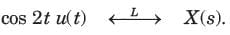Q. Y (s) = ( s + 1) X ( s)

Detailed Solution for Test: Laplace Transform- 3 - Question 5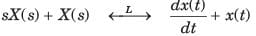⇒ y(t) = ( -2 sin2 t + cos 2 t ) u( t)

Test: Laplace Transform- 3 - Question 6

Given the transform pair below. Determine the time signal y(t) and choose correct option.Q. Y (s) = X ( 3s)

Detailed Solution for Test: Laplace Transform- 3 - Question 6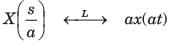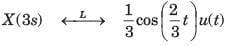Test: Laplace Transform- 3 - Question 7

Given the transform pair below. Determine the time signal y(t) and choose correct option.Q. Y (s) = X ( s + 2)

Detailed Solution for Test: Laplace Transform- 3 - Question 7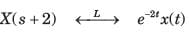Test: Laplace Transform- 3 - Question 8

Given the transform pair below. Determine the time signal y(t) and choose correct option.Q.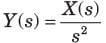Detailed Solution for Test: Laplace Transform- 3 - Question 8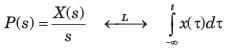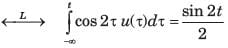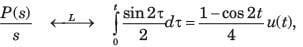Test: Laplace Transform- 3 - Question 9

Given the transform pair below. Determine the time signal y(t) and choose correct option.Q.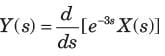Detailed Solution for Test: Laplace Transform- 3 - Question 9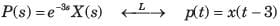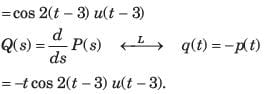Test: Laplace Transform- 3 - Question 10

Given the transform pair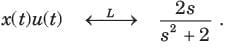Determine the Laplace transform Y (s) of the given time signal in question and choose correct option.
Q. y(t) = x(t - 2)

Detailed Solution for Test: Laplace Transform- 3 - Question 10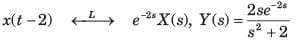## GATE ECE (Electronics) 2023 Mock Test Series

20 docs|263 tests
 Use Code STAYHOME200 and get INR 200 additional OFF Use Coupon Code
Information about Test: Laplace Transform- 3 Page
In this test you can find the Exam questions for Test: Laplace Transform- 3 solved & explained in the simplest way possible. Besides giving Questions and answers for Test: Laplace Transform- 3, EduRev gives you an ample number of Online tests for practice

## GATE ECE (Electronics) 2023 Mock Test Series

20 docs|263 tests